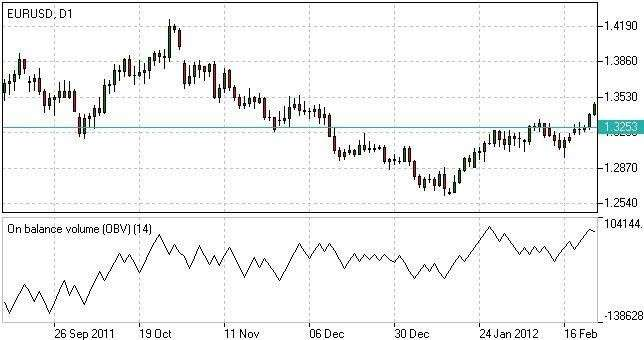# ON-BALANCE VOLUME

On-Balance volume is a volume-dependent tool meant to reveal the connection of deals and asset’s price movements. This is used to confirm a trend and reversals.

When charts show a rising OBV together with decreasing prices, it denotes unstable downtrend ready to turn into a bullish reversal. When OBV falls together with rising prices, the uptrend is weakening and there is a possibility of bearish reversal.

Once the line rises, a price uptrend is verified. When the line falls, a price downtrend is confirmed.

FORMULA

OBV(t) = OBV(t-1) + Vol, if C(t) > C(t-1);

OBV(t) = OBV(t-1) – Vol, if C(t) < C(t-1);

OBV(t) = OBV(t-1), if C(t) = C(t-1),

where:

t – current period;

t-1 – previous period;

C – close price;

Vol – volume.